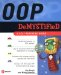# Chapter 7

1. An attribute is information used to describe an object, whereas data is the smallest amount of information used to describe an object. For example, a student name is an attribute, and a students first name and last name are data.

2. A leveling diagram is used to simplify the understanding of a complex application. The first level of the leveling diagram provides a simplified overview of the application, and subsequent levels provide a progressively more detailed view of the application.

3. Entity is another term used for object.

4. The purpose of using an entity relationship diagram is to illustrate the functional relationship between two or more entities that are not hierarchically related to each other.

5. 0:N is a ratio used in an entity relationship diagram to define a relationship. For example, 0:N in a student course relationship means that a student doesnt have to register for any course and could register for many courses.

6. Decomposing an attribute is the process of reducing an attribute to data.

7. A processing model is a diagram that illustrates the steps in a behavior of an object.

8. Pseudo code is a textual description of a behavior that uses a combination of English words and programming language syntax.

9. A class diagram is an illustration that describes a class, its attributes, and its behaviors.

10. A many-to-one relationship defines the relationship between two entities where there are many instances of one entity to one instance of another. For example, many students are in one course.OOP Demystified
ISBN: 0072253630
EAN: 2147483647
Year: 2006
Pages: 130

Similar book on Amazon